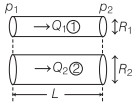# Rate of Flow of Liquid | Methods – Hydrodynamics

## Rate of Flow of Liquid | Methods – Hydrodynamics

We are giving a detailed and clear sheet on all Physics Notes that are very useful to understand the Basic Physics Concepts.

Rate of Flow of Liquid:
Rate of Flow of Liquid through a capillary tube is given by

v = $$\frac{p}{R}$$

where,
R = $$\frac{8 \eta l}{\pi r^{4}}$$ called liquid resistance and
p = liquid pressure.

(i) When two tubes are connected in series:(a) Resultant pressure difference,

p = p1 + p2.

(b) Rate of flow of liquid (v) is same through both tubes.
(c) Equivalent liquid resistance,

R = R1 + R2.

(ii) When two tubes are connected in parallel:(a) Pressure difference (p) is same across both tubes.
(b) Rate of flow of liquid v = v1 + v2.
(c) Equivalent liquid resistance = $$\frac{1}{R}=\frac{1}{R_{1}}+\frac{1}{R_{2}}$$

Hydrodynamics:
In physics, hydrodynamics of fluid dynamics explains the mechanism of fluid such as flow of liquids and gases. It has a wide range of applications such as evaluating forces and momentum on aircraft, prediction of weather, etc.